# SAT Math Multiple Choice Question 74: Answer and Explanation

### Test Information

Question: 74

14.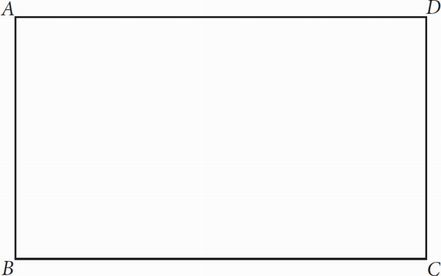If rectangle ABCD has an area of 324 and the tangent of ∠BCA (not shown) is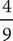, then which of the following is closest to the length of BD (not shown)?

• A. 9.8
• B. 27
• C. 29.5
• D. It cannot be determined from the given information.

CFirst draw lines AC and BD. Now, since tangent is opposite over adjacent,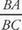=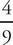. Also, BA × BC = 324. Using these two equations as a system of equations can now help get what is needed. Rearrange the first equation by multiplying both sides by BC to get BA =BC. Now substituteBC into the first equation to getBC (BC) = 324; this simplifies toBC2 = 324. Multiply both sides by (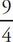) to get BC2 = 729, and then take the square root to get BC = 27. Since the diagonal has to be larger than any of the sides, (A) and (B) are out. Choice (D) can also be eliminated because our previous calculations can get the length of DC, which is used in the Pythagorean theorem to get BD. Therefore, the correct answer is (C).